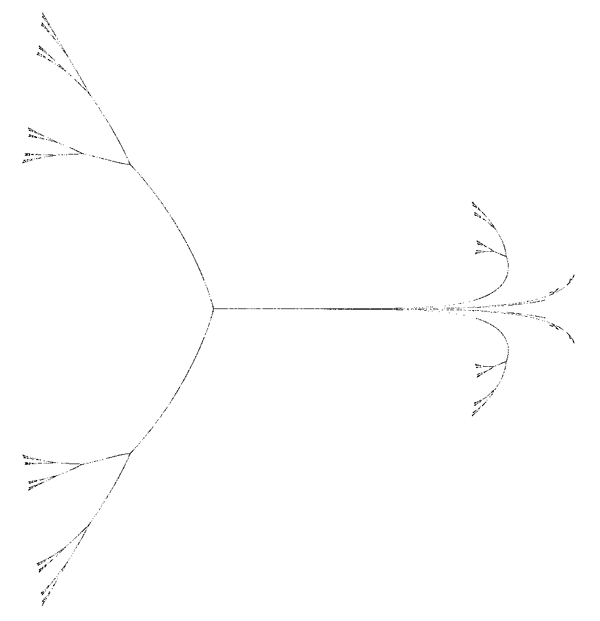# Measure function example

By Roger Bagula
Compiled and graphics by Paul Bourke
6 July 1998I will give you an example of a function that is useful developed from measure ideas. Suppose we have a measure:

m(x,y) = x^s + y^s

and another measure:

m2(x,y) = sqr(x^2 + y^2)

and we try to get a function where both measures are true in the limit domain 0>s>2:

f(x,y,s) = x / (x^s + y^(2 * (s - 1)))^(1/s)

g(x,y,s) = y / (x^s + y^(2 * (s - 1)))^(1/s)

f^s + g^(2 * (s - 1)) = 1

Such a function has some simplicity and theoretical worth and can be understood by almost everyone as a demonstration of what measures mean geometrically. If we change it into an iteration function:

x = x / (x^s + y^(2 * (s - 1)))^(1/s) + a(i)

y = x / (x^s + y^(2 * (s - 1)))^(1/s) + a(i)

and let:

s = abs(abs(cos(atan(y / x)) - 0.1) : 0.1 <= s <= 0.9

we, then, have a visualization of a measure function! It is a connected function that is very probably self-affine and very near d = 1.